# E-PolyLearning

 1. Three piping networks as shown in the figure are placed horizontally. They are made using identical pipe segments and are subjected to the same pressure drop across them. Assuming no pressure losses at junctions, the flow rates across the three networks are related as Q₁ : Q₂ : Q₃.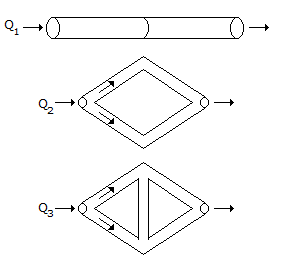a. 1:√3:2 b. 1:2:3 c. 1:2:2 d. 1:√2:√2

 2. For flow through an orifice from a reservoir, the actual velocity at the vena con-tracta is given by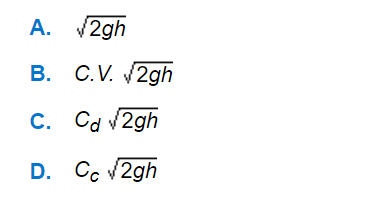a. A b. B c. C d. D
 3. Which of the following is the 'Blaussius equation', relating friction factor to the Reynolds number ?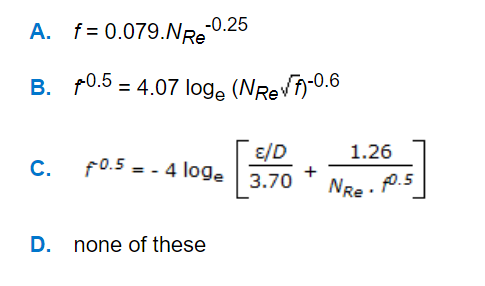a. A b. B c. C d. D
 4. The energy equation, given by following equation, where (E = internal energy/mass), is applicable to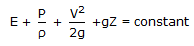a. perfect gases only. b. isothermal flow of gases. c. adiabatic unsteady flow of gases. d. all compressible fluids.
 5. What is the ratio of fluid carrying capacity of two pipes having diameters d₁ and d₂ respectively ?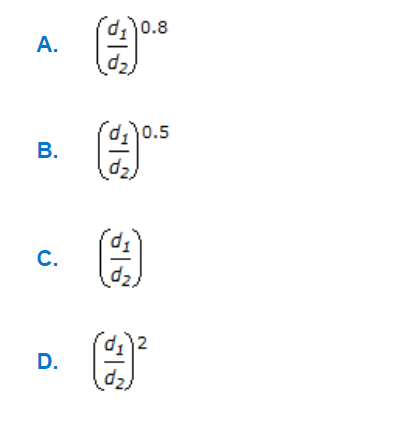a. A b. B c. C d. D